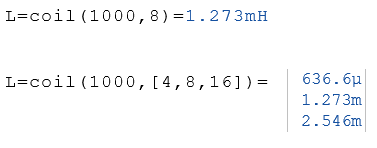# Coil Function

Calculates a coil for the given frequency and impedance

## Calculate a coil

The Coil function calculates a coil for the given frequency and impedance. The parameters for the frequency f and the impedance Z can be real numbers or lists of real numbers.

Coil (f, Z)

### Legend

 f Frequency in Hz Z Impedance in Ohm

### Example